telescopeѲptics.net          ▪▪▪▪                                             CONTENTS

10.2.1.3. Honders camera       10.2.2.1. Schmidt camera: aberrations

10.2.2. Full-aperture Schmidt corrector: Schmidt camera

The simplest arrangement using full-aperture corrector is a camera, with the only other optical element required being a single concave mirror. By far the most popular arrangement is the Schmidt camera.

Back in 1930, Estonian-born optician Bernhard Schmidt succeeded in designing and making full-aperture corrector for spherical mirror. It resulted in a highly corrected optical system, known as Schmidt camera. Somewhat earlier, in 1924, Finnish astronomer Y. Vaisala, described similar arrangement, so this type of camera is sometimes called Schmidt-Vaisala (usually when incorporating field-flattener). Its concept is based on the unique property of spherical mirror with the aperture stop at the center of curvature to be free from off-axis aberrations. The only image aberration remaining is spherical, and it can be cancelled by appropriately figured lens corrector, placed at the mirror center of curvature. The lens element - called Schmidt corrector - has very shallow aspheric curve calculated to give to the incoming wavefront just the needed amount of deformation to result in spherical reflected wavefront (FIG. 167). The only significant aberration induced by the Schmidt corrector is its corrective spherical aberration, resulting in a system free of four primary aberrations: spherical, coma, astigmatism and distortion. The only remaining aberration is field curvature.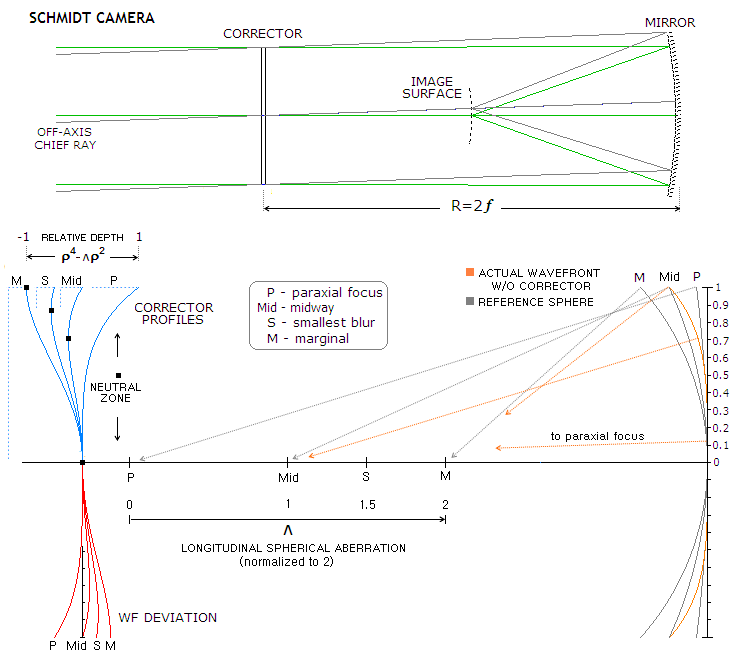FIGURE 167: Schmidt camera (top) is a simple arrangement with the Schmidt corrector at the center of curvature of spherical mirror. Image surface is a curved Petzval surface, concentric with the mirror surface, thus of Ri=R/2 curvature radius. With the aperture stop at the center of curvature, but w/o corrector, the only remaining point-image aberration of the mirror is spherical aberration, causing reflected wavefront (WF) to deviate from spherical by bowing inward excessively toward the edges (spherical undercorrection). It results in the longitudinal aberration, with rays toward outer zones focusing increasingly closer to the mirror. Marginal point of the actual wavefront belongs to a sphere centered at the marginal focus, its paraxial points to a sphere centered at the paraxial focus, and its 0.707 zone point to a sphere centered at the mid-point in between the two. Axial separation between paraxial and marginal focus equals longitudinal defocus. For ease of calculation, it is normalized to 2, zero being at the paraxial focus, and 2 at the marginal focus.
The role of the Schmidt corrector is to modify the incident flat wavefront, so that after reflection from the mirror it becomes spherical, directing all rays to a single point. In effect, the lens compensates for the optical path difference created at the mirror surface, and the corrected wavefront coincides with the corresponding reference sphere. For that, the corrector's profile has to be the inverse of the profile of the aberrated wavefront (bottom left, red), modified by the light speed differential in given mediums (usually air and glass), i.e. deeper by a factor 1/(n'-n), n', n being the the refractive index of the exit and entry medium, respectively. For air and common crown, the profile is about twice deeper than the aberrated wavefront, and for mirror surface it is only half as deep.
Ray paths from every point at this modified wavefront bring points in phase at the sphere centered at the corrected focus, determined by the shape of the corrector profile. Shown are four out of many possible shapes of the Schmidt corrector surface (rear side), greatly exaggerated (bottom left, blue). From left to right, neutral zone (NZ) - the zero slope zone with zero refraction and zero relative wavefront retardation - is at ρ=1,
0.75, 0.5 and 0 of the corrector aperture radius normalized to ρmax=1.
Relative depth of the corrector's curve, ρ
4-
Λρ2, as well as its shape - varies with its focus parameter Λ (0≤Λ≤2), which determines the corrected focus location within longitudinal mirror defocus normalized to 2 (zero being at the paraxial focus, and 2 at the marginal focus, with the value of ρ being the normalized zonal height (0≤ρ≤1). The value of ρ giving the maximum relative depth equals the relative height of the neutral zone: 0, 0.50.5, 0.750.5 and 1, for the corrected focus coinciding with paraxial, best, smallest blur and marginal focus location, respectively. The value of focus parameter Λ and the position of neutral zone NZ in terms of corrector's normalized radius are related as NZ=(Λ/2)1/2ρmax.

Expectedly, with the Schmidt corrector for primary spherical aberration, there is a direct connection between the Schmidt curve and parabolizing. The most efficient mirror parabolizing method is working the center and the edges of a sphere the most, gradually reducing glass removal to a minimum at the 0.707 zone. This surface modification causes relative advance of the wavefront that culminates at the 0.707 zone, and diminishes to zero at the edge and the center, resulting in a corrected, spherical shape of the wavefront. This same wavefront modification is accomplished by placing the neutral zone at 0.707 radius of the Schmidt corrector (in fact, the curve of change of a spherical surface in parabolizing is of the same type as the curve polished into a Schmidt corrector, only shallower). Consequently, for a given mirror, the theoretical maximum thickness of glass needed to be removed from mirror center and the edge, when parabolizing, is smaller by a factor of (n-1)/2 from the (maximum) Schmidt corrector depth at the 0.707 zone (when corrected for primary spherical alone; adding higher order terms makes corrector slightly deeper, with the edge slightly raised vs. center). This holds true for any corrector/parabola pair with identical final focus location. In either case, the volume of glass needed to remove is in inverse proportion to the 3rd power of relative aperture (F-number) of the corrected surface.

Knowing that spherical reflecting surface produces wavefront that in the first term, according to Eq. 4.6, advances away from spherical at a rate of (ρd)4/4R3 with respect to the reference sphere centered at paraxial focus, variation in the Schmidt surface zonal depth z, i.e. surface profile needed for pre-correction of primary spherical aberration that will bring all reflected rays to paraxial focus is z=(ρd)4/4(n-1)R3. This adds the compensatory optical path length (n-1)z=(ρd)4/4R3, which pre-deforms the wavefront, so that it in effect is then corrected by the aberration generated at the mirror. Since the actual wavefront deviation depends on the reference sphere, i.e. specific focus within the aberration longitudinal range to which the rays are to be brought, corrector sag also depends on the normalized defocus Λ, which determines the reference point. The actual wavefront deviation from the reference sphere centered on the chosen zonal focus also varies with the zonal height. Consequently, corrector's depth profile needed for corrected mirror's lower-order spherical aberration also varies with zonal height, as given with the general relation: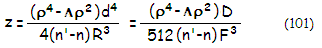where Λ is the relative focus location parameter (from Λ=0 for the corrected focus coinciding with paraxial focus, to Λ=2 when corrected focus coincides with marginal focus), ρ the height in the pupil normalized to 1, d and D the pupil (aperture) radius and diameter, respectively, n the glass index of refraction, n' the index of refraction of the incident/exit media (media next to the Schmidt surface, normally air, with n'=1 for the rear, and n'=n for the front), R the mirror radius of curvature and F the mirror focal ratio for the clear (corrector) aperture. Corrector's focus parameter Λ determines neutral zone location at the unit radius as NZ=(Λ/2)1/2, as well as corrector's aspheric coefficient b; the two determine the needed vertex radius of curvature of the positive central section of the corrector lens Rc.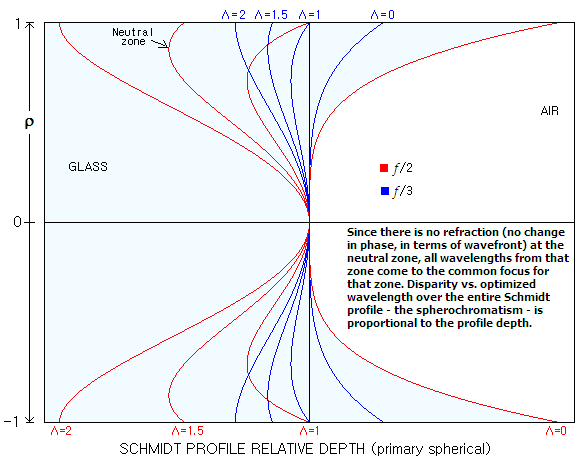Thus, as shown at left, choice of Λ determines the Schmidt profile shape, while its depth varies in proportion to the stop aperture diameter D, and inversely to the third power of mirror's stop-aperture focal ratio F. Any of these profiles has a higher-order aspheric form, which by altering the wavefront form generates spherical aberration of the  magnitude, type and sign needed to offset that of the mirror. As plots show, the strength of corrector's vertex radius of curvature changes inversely to the focus factor Λ, decreasing from infinity for Λ=0 (the profile bringing together the paraxial foci of all wavelengths) to its minimum value for Λ=2 (the profile bringing together marginal foci of all wavelengths). According to NZ=(Λ/2)1/2, neutral zone location shifts from 0 to 1. Profile depth is at its minimum for Λ=1, bringing together best foci of all wavelengths. This profile also induces the least amount of spherochromatism, which is proportional to the profile depth. The profiles shown are based on Eq. 101, hence they only correct for primary spherical. However, even the first next higher order is only a small fraction of the primary spherical, thus the inclusion of higher order terms result only in minor profile modifications (note that the profiles are grossly exaggerated for clarity; actual profiles are seldom more than a few hundreds of a millimeter deep).

Expectedly, the Schmidt surface profile is effectively the shape of the wavefront deviation given by Eq. 7, modified by the 1/(n'-n) medium factor (the profile is opposite in sign to that of the P-V wavefront deviation for the rear corrector's surface, and of the same sign for the front surface). The Λ factor merely determines the amount of defocus with which the paraxial spherical aberration combines in producing the corresponding wavefront for a specific point of defocus.

Alternately, Eq. 101 can be written in terms of the corrector's glass thickness, as t=t1+z, with t1 being the corrector center thickness (mirror radius R is numerically negative, and the sign of z is determined by ρ4-Λρ2, which is always positive for Λ=0, positive for smaller values of ρ and negative for larger ones for 0<ρ<1, and always negative for Λ≥1).

The relative depth of corrector's curve, in units of the maximum corrector depth for 0<Λ<2 is given by ρ4- Λρ2. According to it, depth of corrector's curve is smallest for Λ=1, i.e. with the neutral zone placed at (Λ/2)1/2=0.707 radius (thus ρ=0.707), with the corrected focus coinciding with best focus location (0.866 radius neutral zone placement, with the corresponding Λ value of 1.5, requires corrector deeper by a factor of 2.25). This neutral zone position - as it will be explained in more details ahead - also minimizes spherochromatism.

Most often, at least one higher-order term is significant and needs to be corrected as well. In such case, corrector's curve depth profile can be expressed in terms of its vertex radius of curvature and aspheric values. With the term for higher-order (secondary) spherical aberration added, it is given as :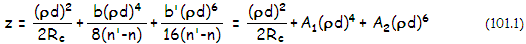with Rc being the corrector vertex radius of curvature, b and b' the 3rd and 5th order aspheric coefficient (for the transverse ray aberration; 4th and 6th order on the wavefront), with A1 and A2 being the corrector's aspheric parameters for the primery and secondary spherical aberration, respectively, commonly used in ray tracing programs. The A1 term - the primary spherical aberration term - is directly related to the conic K as A1=Kd4/8R3, R being the mirror radius of curvature. Note that the first term, sometimes referred to as a2 (with the next being a4, a6 and so on) is in the parabolic form because d/Rc is negligibly small in the full expression for the first term given by d2/R{1+[1-(1+K)(d/R)2]1/2}, with K being the surface conic.

The first term describes sagitta of the corrector's radius of curvature which, combined with the aberration terms (the second is for primary spherical, the third for secondary spherical, and so on), determines the actual surface profile (FIG. 168, left). It is not an aberration term with respect to spherical aberration in the optimized wavelength, since it is corrected for any corrector shape, but it does affect correction of unoptimized wavelengths, i.e. magnitude of spherochromatism. This first term, often called radius term, is actually defocus term: analogous to the aberration terms Ai, which are the wavefront functions of spherical aberration for paraxial focus, it represents defocus aberration with which spherical aberration combines producing altered wavefront specific to any point of defocus, as a sum of the wavefront errors of defocus and spherical aberration.

Obviously, since the required surface profile is directly determined by that of the aberrated wavefront to correct and the medium in which light travels, this term - representing the defocus P-V wavefront error - is is also modified by the same 1/(n'-n) factor.

The second and third term are the P-V wavefront error of primary and secondary spherical at paraxial focus, respectively, modified by the 1/(n'-n) medium factor. Note that only the second term - primary spherical - effectively combines with the defocus (radius) term. Secondary spherical is added only as the P-V wavefront error at paraxial focus, which means that secondary spherochromatism is not minimized. Considering usually small magnitude of secondary spherical, this is negligible; however, in fabrication it is generally more convenient to use the term for minimized secondary spherical, with (ρd)6 replaced by (ρ62)d6. It gives a profile very similar to that for the primary spherical at the best focus (i.e. for Λ=1 and NZ=0.707), which is of the same sign, only much smaller in magnitude. This means that the profile needs to be only slightly deeper at the 0.7 zone, with the change in depth diminishing to zero at the center and the edge, as opposed to having to make the entire corrector deeper by 2.6 times more (FIG. 168 bottom right) than the required deepening at the 0.7 zone.

Hence the addition of higher-order terms requires modifying the profile shown on FIG. 167. Since the Schmidt surface profile is essentially the reversed shape of the wavefront deviation, only deeper by a factor 1/(n-1), n being the glass refractive index, the new profile is a reversed (if on the front surface, same orientation as wavefront if on the back) stretched out in depth replica of the wavefront deviation, as illustrated on FIG. 168 right.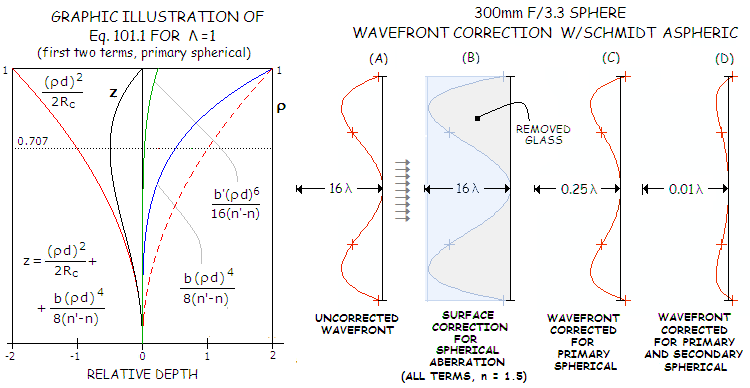FIGURE 168
: TOP: LEFT -- The value of R
c (radius of curvature over the central portion of the corrector) results from a sum of two wavefront deviations: that of spherical aberration at paraxial focus, and that of defocus (opposite in sign) applied to it. This sum is then corrected (enlarged) by the 1/(n'-n) medium term - n being the glass refractive index - to determine the shape of Schmidt corrector. Being the index-corrected sum of two wavefront deviations - that of spherical aberration at paraxial focus, and the defocus wavefront - the implicates the defocus term applied, and vice versa. With the first aberration term only (primary spherical) and Λ=1 (best focus location), the sum is that of the red plot (defocus wavefront deviation) and blue plot (spherical aberration at paraxial focus), resulting in the z plot, which is the needed surface profile before correction by the 1/(n'-n) glass index factor. It can be written as (d4/2R3)(ρ4-ρ2), i.e. it is proportional to ρ4-ρ2, with the neutral (reversal) zone at 0.707 pupil radius. It is easy to see that for Λ=0 (bringing rays to paraxial focus) Rc is infinitely large, the first term (i.e. defocus error) is zero, and it is the second, spherical aberration term alone (blue) that determines corrector's profile, effectively the inverse of the wavefront deviation at paraxial focus multiplied by 1 (n'-n). The relative dept - or dimensionless profile of the corrector (FIG. 167 left) - for corrected primary spherical alone is given by  ρ4- Λρ2. Adding the next, higher-order term, for secondary spherical (green, grossly exaggerated for clarity) raises the edge vs. inner area, practically requiring deepening the corrector evenly for the value of the term from center to 0.7 zone, and gradually raising toward the old profile line and unchanged edge from the 0.7 zone up. More practical way of fabricating such corrector is adding secondary spherical at the best focus location (bottom right).  RIGHT - Grossly exaggerated lens surface deformation (B) needed to correct spherical aberration (A) of a 300mm f/3.3 spherical mirror, with the glass index of refraction n=1.5. Most of the 7.6 wave P-V aberration is its primary term, with the remaining higher-order terms totaling less than 1/10 wave P-V (C). Maximum depth of the aspheric curve for correcting the primary term is, therefore, about 7.5 waves times 1/(n-1), or 15 waves for n=1.5. For correcting the secondary spherical, less than 0.2 wave deeper curve is needed, with the remaining residual higher order term (tertiary spherical) of less than 0.002 wave P-V (D). Higher order terms grow exponentially with the relative aperture, the higher term, the faster.
MIDDLE: Illustration of the parameters shaping up the Schmidt profile for the two common shapes (neutral zone at 0.707 and 0.866 radius), and adding correction for secondary spherical. As before, both, pupil radius and primary spherical aberration coefficient normalized to 1. Each term - for defocus (P), primary (A4) and secondary (A6) spherical - is proportional to the corresponding aberration function for P-V wavefront error. The defocus aberration function - i.e. the normalized wavefront error of defocus with reference to the paraxial focus - is the radius term, ranging from 0 to -2, in units of the P-V wavefront error of primary spherical at paraxial focus.
LEFT: The P-V wavefront error of spherical aberration at paraxial focus, green (determines A4, proportional to ρ
4), and defocus
P-V WFE, proportional to ρ
2 and 1.5ρ2 (blue and red, for defocus at the best focus location and smallest blur, respectively). Zero defocus is a, leaving the wavefront shape at the paraxial focus unaltered, best focus defocus is b (not to be confused with the primary spherical aspheric coefficient b), and the smallest blur location defocus is c.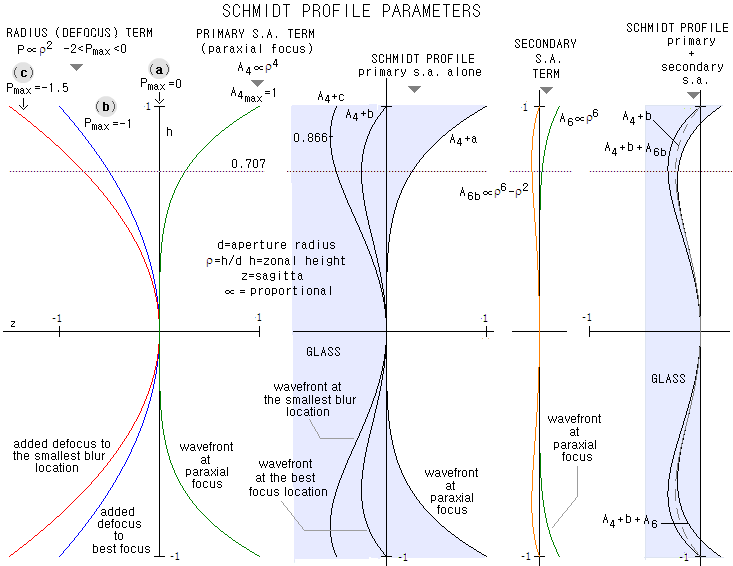SECOND FROM LEFT: The resulting profile shapes after adding zero (A4+a), best focus (A4+b, neutral zone at the 0.707 radius height) and the smallest blur point defocus  (A4+c, neutral zone at 0.866 radius) to the P-V WFE of s.a. at paraxial focus.
THIRD FROM LEFT: The P-V wavefront error of secondary spherical to be added for correcting the secondary spherical, for the paraxial (determines A6, proportional to ρ
6) and best focus (proportional to ρ62) location (note that ρ62 is best focus with respect to the P-V error; for the minimum RMS error, best focus requires a bit less of defocus from the paraxial focus,
and is given by ρ
6-0.9ρ2).
RIGHT: The sum of wavefront deviation profiles for primary spherical and defocus with the secondary spherical wavefront deviation profile added. The conventional profile relation adds the secondary term for paraxial focus, proportional to ρ
6, but for fabrication purposes the best focus term, proportional to ρ62, is preferable, requiring shallower corrector with the center and edge in the same plane. Graph below illustrates more clearly the difference between two profiles. A profile balancing lower and higher order term (i.e. adding as much of the lower order as needed to minimize the higher order, but without entirely correcting the latter) is very close to the profile entirely correcting for the 6th (and 4th) order, indicating high degree of accuracy needed to fully correct for the higher-order term; however, it being usually low, reducing it to a small fraction is, for all practical purposes, cancelling it out.
Note that the actual glass profiles depths z are larger by a factor 1/(n'-n) than the functions representing the sum of primary spherical and given amounts of defocus shown (normalized to 1 for the P-V wavefront error of mirror spherical aberration at the paraxial focus).

BOTTOM: Shapes of the Schmidt profile for correcting primary spherical at the best focus alone, primary and secondary spherical at either its paraxial or best focus location, and for zero secondary spherical coefficient (A6), with the needed amount of primary spherical added to balance (minimize) secondary spherical.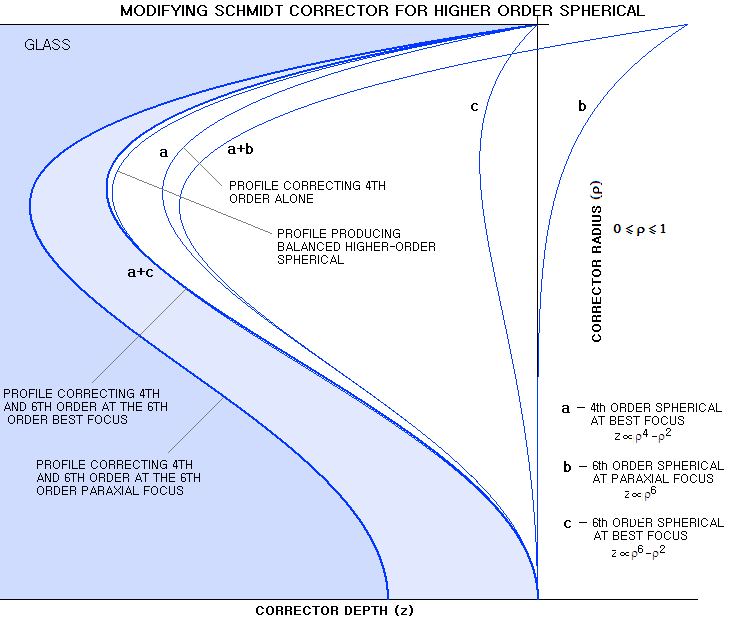Pure secondary spherical (c and b) is exaggerated vs. primary spherical, in order to have different profiles clearly separated. The wavefronts generated by these profiles would have the same form of deviation, only shallower by a factor (n-1), n being the glass refractive index. Profile correcting for primary spherical (i.e. 4th order) alone (a) leaves secondary spherical (6th order) uncorrected, with the smallest error being at its respective best focus. Profile that minimizes this residual secondary spherical by adding primary spherical of opposite sign is only slightly different than the fully correcting profile, and in all but very strong correctors it would be beyond fabrication accuracy to opt for one or the other.
For instance, the secondary spherical residual in an 8 inch commercial SCT is about 1/8 wave P-V at the paraxial focus, and 2.5 times less at the best focus. Since the latter is reduced over four times when offset with opposite primary spherical, the differential between full correction is less than 1/80 wave in the wavefront, and about twice as much in the glass profile.
Profile given with Eq. 101.1 fully corrects for secondary spherical at its paraxial focus. This, however, is less practical profile from the fabrication point of view, since adding profile correction for the secondary spherical at paraxial focus (b) to the profile for primary spherical at best focus (a) results in a deeper profile with uneven center/edge height (since glass cannot be added, this profile, marked a+b, is pulled into the glass so that its edge coincides with the glass edge). It is generally easier to produce profile correcting for secondary spherical at its best focus (a+c) which, as mentioned, is often nearly identical to the profile that only minimizes secondary spherical (in such case, it is reasonable to assume that the 6th order term, as given in Eq. 101.1, is dropped, and only 4th order term, corrected for the amount needed to minimize the secondary spherical residual, is used).

Schmidt surface for correcting spherical aberration of a conic surface has all its surface terms of the same sign which, according to Eq. 101.1, implies that correcting the next order term requires deeper surface profile. More complex forms of spherical aberration, such as, for instance, correcting balanced higher order forms (Maksutov corrector, strongly curved refracting objectives, and other) may have higher surface terms of different numerical sign, where correcting next higher order term may require shallower curve. In principle, there is no difference in the effect of aspheric profile whether it is applied to a flat surface, or radius (the latter merely requires adjustment for the corrector's radius when entering specs into raytrace).

The Schmidt corrector radius of curvature is given by: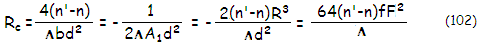with the 3rd order aspheric coefficient b=2/R3, and n'=1 for the aspheric surface on the back of corrector ( is the mirror focal length, and F the focal ratio). Optionally, the relations can be written in terms of the relative neutral zone position in units of aperture radius, NZ, by substituting Λ=2NZ2. Optimized for the small effect of corrector's radius of curvature, 3rd order aspheric coefficient is: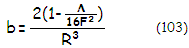with F being the mirror F-number (F=-R/2D). The 5th order aspheric coefficient b'=6/R5. The two aspheric parameters  A1 and  A2 determine the Schmidt corrector shape, according to Eq. 101.1. From the equation, they are obtained from their respective aspheric coefficients b and b', as

A1 = b/8(n'-n) = 1/4(n'-n)R3           (104)  and

A2 = b'/16(n'-n) = 3/8(n'-n)R5           (104.1)

The two aspheric coefficients, b and b', are obtained by setting the system aberration coefficients for 3rd and 5th order spherical aberration to zero, s3=-b/8 + [1-(Λ/16F2)]/4R3 = 0 and s5=-b'/16 + 3/8R5 = 0 with the left side of the coefficient (b factor) being the corrector aberration contribution, and the right side that of the mirror. The 4th and 6th order system P-V wavefront error at the paraxial focus are W3=s3d4 and W5=s5d6, respectively.

The slightly lower 3rd order mirror coefficient results from its effective relative aperture slightly reduced for non-zero values of corrector's focus parameter Λ (in effect, the higher Λ, the more diverging outer rays falling onto mirror, reducing spherical aberration). A non-zero paraxial radius term Rc makes the corrector a weak positive lens with aspheric figure, also determining neutral zone position for given value of the aspheric coefficient b. The neutral zone location is also given directly, for unit radius, as NZ=(Λ/2)1/2.

The significance of the 5th order term is in correction of the higher-order spherical aberration (5th order transverse ray, 6th order on the wavefront). Those include axial spherical, as well as oblique (lateral) spherical, and wings, the higher-order astigmatism as it was named by Schwarzschild. They both increase with the square of off-axis height in the image space, and set the limit to field quality. The latter has the P-V error larger by a factor of 4n, n being the glass refractive; since it varies with cosθ, θ being the pupil angle, the off-axis aberration in the Schmidt camera peaks along the tangential plane (the one determined by the chief ray and optical axis, for which θ=0 and cosθ=1).

For 200mm f/2 Schmidt camera, the amount of higher-order spherical aberration is ~0.24 wave RMS. It can be minimized by balancing it with the lower-order form of opposite sign (by making the 4th order curve slightly stronger). The residual that can't be corrected with the 3rd order surface term alone is ~0.04 wave RMS.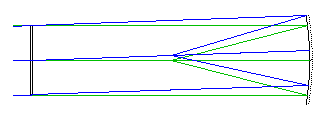EXAMPLE: 200mm f/2 Schmidt camera with BK7 corrector (n=1.5185 for 550nm wavelength), thus clear aperture radius d=100 at the corrector, and mirror radius of curvature R=-800. Choosing for the corrected focus best focus location of the mirror, thus Λ=1, determines neutral zone height NZ=(Λ/2)1/2ρmax, at 0.707d. Only the rear side is aspherized.

From Eq. 103, corrector's lower-order aspheric coefficient b=2[1-/16F2)]/R3=-0.000000003845, or b=-3.845-9, determining the lower-order aspheric parameter of the corrector as A1=b/8(n'-n)=9.27-10, with the index of refraction of the exit media (air for the Schmidt surface at the back of corrector) n'=1. Higher-order aspheric coefficient b'=6/R5=-1.83-14 determines the higher-order corrector aspheric parameter A2=b'/16(n'-n) =2.21-15. Needed radius of curvature of the corrector is Rc=-1/2ΛA 1d2=-53,940mm.

With the corrector at the mirror center of curvature, the system is corrected for 3rd/4th i.e. 5th/6th order spherical aberration (3rd and 5th transverse ray aberration, corresponding to 4th and 6th order on the wavefront), coma, astigmatism and distortion. The only remaining aberration is field curvature, rc=R/2=-400mm.

For double-sided corrector, both b and A coefficients are half their value for single-sided corrector, with the A coefficients being of the opposite sign on the other side (as determined by n'-n)..

Since, from Eq. 104/104.1, b=8(n'-n)A1 and b'=16(n'-n)A2, the P-V wavefront error at the best focus resulting from deviations  ΔA1 and  ΔA2 in the two aspheric parameters is given by W4=(n'-n)ΔA1d4/4 and W6=0.42(n'-n)ΔA2d6 for 4th and 6th order spherical aberration, respectively. Taking 0.0001375mm (1/4 wave at 0.00055mm wavelength) for W4 gives, for the above system, the corresponding lower-order parameter deviation as ΔA1=4W4/(n'-n)d4=1.06-11, with 1/4 wave of spherical aberration figure tolerance for the lower-order aberration of 1.06-11(ρd)4. At the maximum curve depth (ρ=0.707), it is 0.000265mm, or 0.48 wave.

Follows more detailed account of the Schmidt camera aberrations.